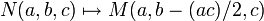Baer correspondence between NT(3,p) and UT(3,p)

Throughout this article,$p$ is an odd prime number.

This article is about a particular instance of a Baer correspondence, and hence also a Lazard correspondence, between a Lie ring and a group, both of order$p^3$ and class two.

Note that most aspects of this article generalize to the following situations:

• We replace$\mathbb{F}_p$ by a finite field$\mathbb{F}_q$ or an infinite field of characteristic not equal to 2.
• Even more generally, we replace$\mathbb{F}_p$ by a ring whose characteristic is not divisible by 2. For instance, we may replace it by$\mathbb{Z}/9\mathbb{Z}$.

The parts that fail to generalize are the precise descriptions of subgroups and endomorphisms: in the more general cases, there are additional subgroups and endomorphisms.

The Lie ring

The Lie ring$NT(3,p)$ is niltriangular matrix Lie ring:NT(3,p). More explicitly, it is the Lie ring whose elements are$3 \times 3$ matrices over the prime field$\mathbb{F}_p$, with 0s on and below the diagonal, i.e., matrices of the form:$M(a,b,c) = \begin{pmatrix} 0 & a & b \\ 0 & 0 & c \\ 0 & 0 & 0 \\\end{pmatrix}, \qquad a,b,c \in \mathbb{F}_p$

The addition is defined as matrix addition and the Lie bracket is defined as$[X,Y] = XY - YX$ where the product is matrix multiplication. Explicitly:

Operation How it's defined (short version) How it's defined (formula)
Addition Matrix addition$\! M(a_1,b_1,c_1) + M(a_2,b_2,c_2) = M(a_1 + a_2,b_1+b_2,c_1+c_2)$
Identity for addition Zero matrix$\! M(0,0,0)$
Negative for addition Negative as matrix$\! -M(a,b,c) = M(-a,-b,-c)$
Lie bracket Commutator as matrices, i.e.,$(X,Y) \mapsto XY - YX$$\! [M(a_1,b_1,c_1),M(a_2,b_2,c_2)] = M(0,a_1c_2 - a_2c_1,0)$

The group

The group$UT(3,p)$ is unitriangular matrix group:UT(3,p). Explicitly, it is the group whose elements are$3 \times 3$ matrices over the prime field$\mathbb{F}_p$ with$1$s on the diagonal,$0$s below the diagonal, and arbitrary entries above the diagonal, i.e., matrices of the form:$N(a,b,c) = \begin{pmatrix} 1 & a & b \\ 0 & 1 & c \\ 0 & 0 & 1 \\\end{pmatrix}, \qquad a,b,c \in \mathbb{F}_p$

The multiplication is matrix multiplication and the identity element and inverse are the usual identity element and inverse. Explicitly:

Operation How it's defined (short version) How it's defined (formula)
Multiplication Matrix multiplication$\! N(a_1,b_1,c_1)N(a_2,b_2,c_2) = M(a_1 + a_2,b_1+b_2 + a_1c_2,c_1+c_2)$
Identity for multiplication Identity matrix$\! N(0,0,0)$
Inverse for multiplication Inverse matrix$\! N(a,b,c)^{-1} = N(-a,ac-b,-c)$

The bijection between the Lie ring and the group

From Lie ring to group

The bijection given by the Baer correspondence is the exponential map for matrices, explicitly:$X \mapsto I + X + \frac{X^2}{2}$

where the addition and multiplication are carried out as matrices. Note that higher powers of$X$ do not appear because$X^3$ becomes zero.

In terms of our notation, this is the map:$\begin{pmatrix}0 & a & b \\ 0 & 0 & c \\ 0 & 0 & 0 \\\end{pmatrix} \mapsto \begin{pmatrix} 1 & a & b + (ac)/2 \\ 0 & 1 & c \\ 0 & 0 & 1 \\\end{pmatrix}$

Or, in our shorthand:$\! M(a,b,c) \mapsto N(a,b+(ac)/2,c)$

From group to Lie ring

The bijection given by the Baer correspondence is the logarithm map for matrices, explicitly:$X \mapsto (X - I) - \frac{(X - I)^2}{2}$

where the addition and multiplication are carried out as matrices. Note that higher powers of$(X - I)$ do not appear because$(X- I)^3$ becomes zero.

In terms of our notation, this is the map:$\begin{pmatrix}1 & a & b \\ 0 & 1 & c \\ 0 & 0 & 1 \\\end{pmatrix} \mapsto \begin{pmatrix} 0 & a & b - (ac)/2) \\ 0 & 0 & c \\ 0 & 0 & 0 \\\end{pmatrix}$

In our shorthand:$\! N(a,b,c) \mapsto M(a,b - (ac)/2,c)$

Central series

Both the Lie ring and the group are nilpotent of class equal to 2. In fact, they both have the lower central series and upper central series coincide, i.e., the center and derived subgroup are the same for the group, and the center and derived subring are the same for the Lie ring. Specifically, we have:$Z(NT(3,p)) = [NT(3,p),NT(3,p)] = \{ M(0,b,0) : b \in \mathbb{F}_p \}$ is an abelian Lie ring whose additive group is a group of prime order.$Z(UT(3,p)) = [UT(3,p),UT(3,p)] = \{ N(0,b,0) : b \in \mathbb{F}_p \}$ is a group of prime order.

Further, under the bijection,$M(0,b,0) \leftrightarrow N(0,b,0)$, so the bijection restricts to an isomorphism on the center with the obvious identification.

Correspondence between subgroups and subrings

The table below lists the five different automorphism classes of subgroups and the corresponding automorphism classes of subrings, with comments on how the bijection works for each of these.

Automorphism class of subgroups of$UT(3,p)$ Automorphism class of subrings of$NT(3,p)$ Order of the subgroup/subring Index of the subgroup/subring Number of conjugacy classes of subgroups = number of orbits of subrings under Adjoint action of group Size of each conjugacy class = Size of each orbit under Adjoint action of group Total number of subgroups = number of subrings Quotient group$\leftrightarrow$ Quotient Lie ring Subnormal depth
trivial subgroup trivial subring 1$p^3$ 1 1 1 the whole group, whole Lie ring 1
center of prime-cube order group:U(3,p) center of niltriangular matrix Lie ring:NT(3,p)$p$$p^2$ 1 1 1 elementary abelian group of prime-square order (and corresponding abelian Lie ring) 1
non-central subgroups of prime order in unitriangular matrix group:UT(3,p) non-central subrings of prime order in niltriangular matrix Lie ring:NT(3,p)$p$$p^2$$p + 1$$p$$p(p + 1)$ -- 2
elementary abelian subgroups of prime-square order in unitriangular matirx group:UT(3,p) elementary abelian subrings of prime-square order in niltriangular matrix Lie ring:NT(3,p)$p^2$$p$$p + 1$ 1$p + 1$ group of prime order (and corresponding abelian Lie ring) 1
whole group whole Lie ring$p^3$ 1 1 1 1 trivial group, trivial Lie ring 0
Total (5 rows) -- -- --$2p + 5$ --$p^2 + 2p + 4$ -- --